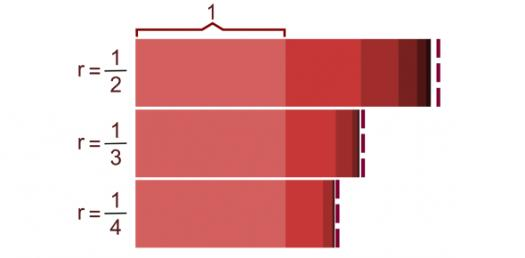# Geometric Sequences Trivia Questions Quiz

5 Questions | Attempts: 233
ShareSettings.

• 1.
Determine which sequence is geometric.
• A.

-16, -13, -10, -7, ...

• B.

1, 1, 2, 3, 5, ...

• C.

-3, -6, -12, -24, ...

• D.

5, 7, 9, 11, ...

• 2.
Find the fifth term in a sequence in which the first term is 3 and the common ratio is -4.
• A.

60

• B.

-3072

• C.

-729

• D.

768

• 3.
What is the 7th term of the geometric sequence:
• A.

-48

• B.

192

• C.

3072

• D.

-12288

• 4.
The 15th term of a geometric sequence is 32,768.  Which choice shows the possible first term and the possible common ratio?
• A.

2, 2

• B.

4, 3

• C.

15, 4

• D.

8, -4

• 5.
Find the next two terms of the geometric sequence 50, 40, 32, ...
• A.

24, 15768

• B.

25.6, 20.48

• C.

26, 22128

• D.

28.5, 25.52

## Related TopicsBack to top
×

Wait!
Here's an interesting quiz for you.